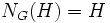# Normalizer condition implies every maximal subgroup is normal

This article gives the statement and possibly, proof, of an implication relation between two group properties. That is, it states that every group satisfying the first group property (i.e., group satisfying normalizer condition) must also satisfy the second group property (i.e., group in which every maximal subgroup is normal)
View all group property implications | View all group property non-implications
Get more facts about group satisfying normalizer condition|Get more facts about group in which every maximal subgroup is normal

## Statement

Suppose$G$ is a group satisfying normalizer condition: it has no proper self-normalizing subgroup. In other words, every subgroup$H$ of$G$ satisfies the property that$H$ is properly contained in its normalizer$N_G(H)$.

Then, any maximal subgroup of$G$ is a normal subgroup.

## Proof

Given: A group$G$ satisfying the normalizer condition, a maximal subgroup$H$ of .

To prove:$H$ is normal in$G$.

Proof:$N_G(H)$ is a subgroup of$G$ containing$H$. Since$H$ is maximal in$G$,$N_G(H) = G$ or$N_G(H) = H$. Since$G$ satisfies the normalizer condition, we cannot have$N_G(H) = H$. Thus,$N_G(H) = G$, and we obtain that$H$ is normal in$G$.